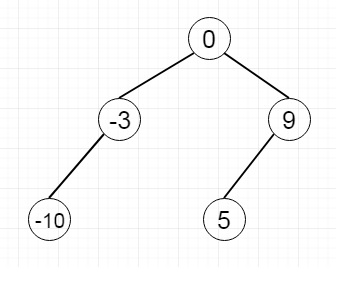# Path Sum in Python

Suppose we have one tree and a sum. We have to find one path such that if we follow that path, we will get the sum that will be matched with the given sum. Suppose the tree is like [0,-3,9,-10, null,5] and the sum is 14, then there is a path 0 → 9 → 5To solve this, we will follow these steps.

• If the root is null, then return False

• if left and right subtree are empty, then return true when sum – root.val = 0, otherwise false

• return solve(root.left, sum – root.val) or solve(root.right, sum – root.val)

Let us see the following implementation to get a better understanding −

## Example

Live Demo

# Definition for a binary tree node.
class TreeNode(object):
def __init__(self, x):
self.data = x
self.left = None
self.right = None
def insert(temp,data):
que = []
que.append(temp)
while (len(que)):
temp = que
que.pop(0)
if (not temp.left):
if data is not None:
temp.left = TreeNode(data)
else:
temp.left = TreeNode(0)
break
else:
que.append(temp.left)
if (not temp.right):
if data is not None:
temp.right = TreeNode(data)
else:
temp.right = TreeNode(0)
break
else:
que.append(temp.right)
def make_tree(elements):
Tree = TreeNode(elements)
for element in elements[1:]:
insert(Tree, element)
return Tree
class Solution(object):
def hasPathSum(self, root, sum):
"""
:type root: TreeNode
:type sum: int
:rtype: bool
"""
if not root :
return False
if not root.left and not root.right and root.data is not None:
return sum - root.data == 0
if root.data is not None:
return self.hasPathSum(root.left, sum-root.data) or self.hasPathSum(root.right, sum-root.data)
tree1 = make_tree([0,-3,9,-10,None,5])
ob1 = Solution()
print(ob1.hasPathSum(tree1, 14))

## Input

tree1 = make_tree([0,-3,9,-10,None,5])

## Output

True